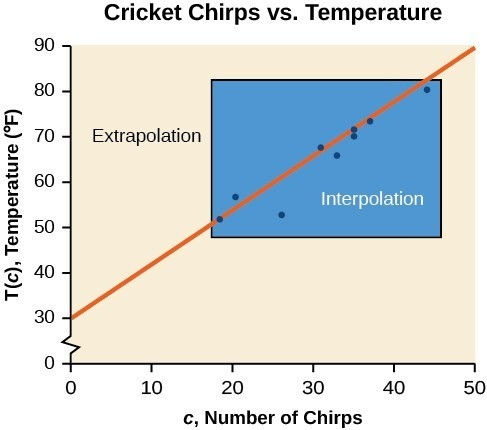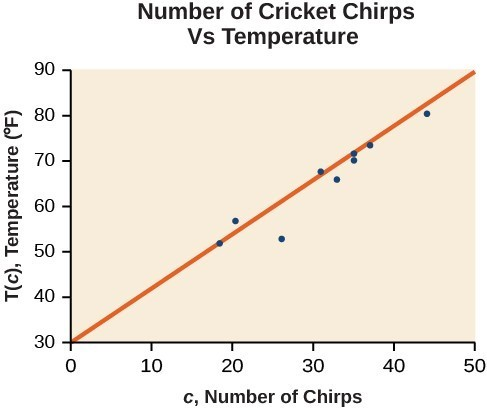## Find the line of best fit

Once we recognize a need for a linear function to model the data in “Draw and interpret scatter plots,” the natural follow-up question is “what is that linear function?” One way to approximate our linear function is to sketch the line that seems to best fit the data. Then we can extend the line until we can verify the y-intercept. We can approximate the slope of the line by extending it until we can estimate the $\frac{\text{rise}}{\text{run}}$.

### Example 2: Finding a Line of Best Fit

Find a linear function that fits the data in the table below by “eyeballing” a line that seems to fit.

 Chirps 44 35 20.4 33 31 35 18.5 37 26 Temperature 80.5 70.5 57 66 68 72 52 73.5 53

### Solution

On a graph, we could try sketching a line.

Using the starting and ending points of our hand drawn line, points (0, 30) and (50, 90), this graph has a slope of

$m=\frac{60}{50}=1.2$

and a y-intercept at 30. This gives an equation of

$T\left(c\right)=1.2c+30$

where c is the number of chirps in 15 seconds, and T(c) is the temperature in degrees Fahrenheit. The resulting equation is represented in the graph below.

### Analysis of the Solution

This linear equation can then be used to approximate answers to various questions we might ask about the trend.

## Recognizing Interpolation or Extrapolation

While the data for most examples does not fall perfectly on the line, the equation is our best guess as to how the relationship will behave outside of the values for which we have data. We use a process known as interpolation when we predict a value inside the domain and range of the data. The process of extrapolation is used when we predict a value outside the domain and range of the data.

The graph below compares the two processes for the cricket-chirp data addressed in Example 2. We can see that interpolation would occur if we used our model to predict temperature when the values for chirps are between 18.5 and 44. Extrapolation would occur if we used our model to predict temperature when the values for chirps are less than 18.5 or greater than 44.

There is a difference between making predictions inside the domain and range of values for which we have data and outside that domain and range. Predicting a value outside of the domain and range has its limitations. When our model no longer applies after a certain point, it is sometimes called model breakdown. For example, predicting a cost function for a period of two years may involve examining the data where the input is the time in years and the output is the cost. But if we try to extrapolate a cost when x = 50, that is in 50 years, the model would not apply because we could not account for factors fifty years in the future.Figure 4. Interpolation occurs within the domain and range of the provided data whereas extrapolation occurs outside.

### A General Note: Interpolation and Extrapolation

Different methods of making predictions are used to analyze data.

• The method of interpolation involves predicting a value inside the domain and/or range of the data.
• The method of extrapolation involves predicting a value outside the domain and/or range of the data.
• Model breakdown occurs at the point when the model no longer applies.

### Example 3: Understanding Interpolation and Extrapolation

 Chirps 44 35 20.4 33 31 35 18.5 37 26 Temperature 80.5 70.5 57 66 68 72 52 73.5 53

Use the cricket data above to answer the following questions:

1. Would predicting the temperature when crickets are chirping 30 times in 15 seconds be interpolation or extrapolation? Make the prediction, and discuss whether it is reasonable.
2. Would predicting the number of chirps crickets will make at 40 degrees be interpolation or extrapolation? Make the prediction, and discuss whether it is reasonable.

### Solution

1. The number of chirps in the data provided varied from 18.5 to 44. A prediction at 30 chirps per 15 seconds is inside the domain of our data, so would be interpolation. Using our model:
$\begin{cases}T\left(30\right)=30+1.2\left(30\right)\hfill \\ \text{ }=66\text{degrees}\hfill \end{cases}$

Based on the data we have, this value seems reasonable.

2. The temperature values varied from 52 to 80.5. Predicting the number of chirps at 40 degrees is extrapolation because 40 is outside the range of our data. Using our model:
$\begin{cases}40=30+1.2c\hfill \\ 10=1.2c\hfill \\ c\approx 8.33\hfill \end{cases}$

We can compare the regions of interpolation and extrapolation using the graph below.

### Analysis of the Solution

Our model predicts the crickets would chirp 8.33 times in 15 seconds. While this might be possible, we have no reason to believe our model is valid outside the domain and range. In fact, generally crickets stop chirping altogether below around 50 degrees.

### Try It 1

According to the data from the table in Example 3, what temperature can we predict it is if we counted 20 chirps in 15 seconds?

Solution

## Finding the Line of Best Fit Using a Graphing Utility

While eyeballing a line works reasonably well, there are statistical techniques for fitting a line to data that minimize the differences between the line and data values. One such technique is called least squares regression and can be computed by many graphing calculators, spreadsheet software, statistical software, and many web-based calculators. Least squares regression is one means to determine the line that best fits the data, and here we will refer to this method as linear regression.

### How To: Given data of input and corresponding outputs from a linear function, find the best fit line using linear regression.

1. Enter the input in List 1 (L1).
2. Enter the output in List 2 (L2).
3. On a graphing utility, select Linear Regression (LinReg).

### Example 4: Finding a Least Squares Regression Line

Find the least squares regression line using the cricket-chirp data in the table below.

 Chirps 44 35 20.4 33 31 35 18.5 37 26 Temperature 80.5 70.5 57 66 68 72 52 73.5 53

### Solution

1. Enter the input (chirps) in List 1 (L1).
2. Enter the output (temperature) in List 2 (L2). See the table below.
 L1 44 35 20.4 33 31 35 18.5 37 26 L2 80.5 70.5 57 66 68 72 52 73.5 53
3. On a graphing utility, select Linear Regression (LinReg). Using the cricket chirp data from earlier, with technology we obtain the equation:
$T\left(c\right)=30.281+1.143c$

### Analysis of the Solution

Notice that this line is quite similar to the equation we “eyeballed” but should fit the data better. Notice also that using this equation would change our prediction for the temperature when hearing 30 chirps in 15 seconds from 66 degrees to:

$\begin{cases}T\left(30\right)=30.281+1.143\left(30\right)\hfill \\ \text{ }=64.571\hfill \\ \text{ }\approx 64.6\text{ degrees}\hfill \end{cases}$Figure 6

The graph of the scatter plot with the least squares regression line is shown in below.

### Q & A

Will there ever be a case where two different lines will serve as the best fit for the data?

No. There is only one best fit line.

1. Technically, the method minimizes the sum of the squared differences in the vertical direction between the line and the data values.
2. For example, http://www.shodor.org/unchem/math/lls/leastsq.html